## Paper & Video

A paper on perspective shadow maps has been accepted for this year's Siggraph. The final version of the paper and a video explaining the technique and showing results can be found here.

## Idea

Shadows are a visually essential lighting detail. Obtaining shadows, however, is usually costly, in particular for dynamic scenes, when shadows change constantly so that no precomputation is possible. A typical approximation for dynamic scenes are thus faked shadows, strongly simplified shadows that are for example drawn on the floor somewhere under the player. This needs to be done for all objects that should cast a shadow, and it becomes difficult if the player is not moving on plane floor. A much more general approach that allows interactive rendering are shadow maps, where the scene is rendered in a first pass from the view of a light source. All points visible in that view are lit. Such shadow maps can be applied to rendered objects using texturing capabilities of modern graphics hardware.

## Examples

The ideal case is depicted in the following image. The viewing frustum (left) becomes a cube in post-perspective space (right). When projecting the shadow map pixels on the ground and then on the image, they all exhibit the same image size, so we have a perfect match between image and shadow map resolution for the ground.Note that this is the ideal case, because the parallel light source remains parallel in post-perspective space. In general, parallel light sources can become point lights in post perspective space and vice versa. Other possible case for parallel light sources are depicted in the following image. Light sources from the front or the back become point lights on the infinity plane in post-perspective space, where the depth ordering is reversed in the letter case.An example for a point (spot) light source is shown in the following images. The upper row shows the light source view for a uniform shadow map (left), its depth buffer (center) and the resulting view (right) with strong aliasing artifacts. The lower row shows the same for our non-uniform shadow map.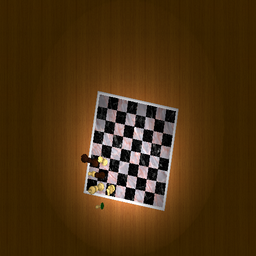uniform shadow map view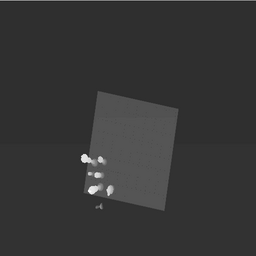uniform shadow map depth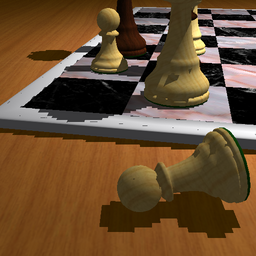uniform shadow map result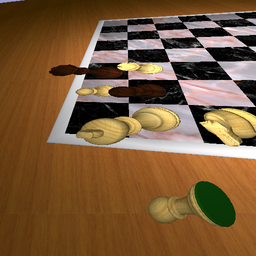perspective shadow map view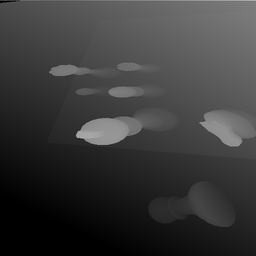perspective shadow map depth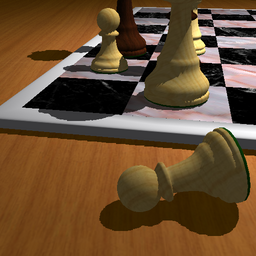perspective shadow map result

## Recipe for Computing the Perspective Shadow Map Matrix

The shadow map is rendered  just as the normal view, with one modelview / projection matrix pair. You  can get that pair as follows: if MV and P are the matrices for the current  camera, then the transformation world space -> post perspective space is the  matrix M=P*MV. This transformation M maps a visible scene point in world space to the unit cube [-1,1]^3. You first have to transform your light source with the same matrix M. If it is a parallel light from (x,y,z) just plug in (x,y,z,0); in general, this point at infinity will end up in a finite point in post-perspetive space. So you have a light source point in post-perspective space and the visible scene is in the unit cube. You then have to setup a normal shadow map for this setting, i.e. a (point) light source, illuminating the unit cube. If the light source is at L you can achieve this with (OpenGL, sorry) gluLookAt(L,L,L,0,0,0,0,0,1), i.e. you put the camera into the light source and look at the origin (center of the unit cube). Choosing z as up-Vector is rather arbitrary. Then you have to compute the perspective opening angle such that the entire cube is visible. This gives you a light modelview matrix MVL and a light projection matrix PL. Thus the entire matrix becomes PL*MVL*P*MV. You can now use PL*MVL*P as new Projection matrix and keep MV as modelview matrix. That's it.

## Gallery